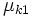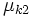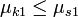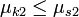# Sliding motion for block piled on block on an inclined plane

(diff) ← Older revision | Latest revision (diff) | Newer revision → (diff)
Consider one block placed on top of another, with the bottom block being$A$ and the block on top being$B$.$A$ is on a fixed inclined plane making an angle of$\theta$ with the horizontal and the surface of contact between$A$ and$B$ is parallel to the inclined plane, i.e., that surface also makes an angle of$\theta$ with the horizontal.
•$\mu_{s1}$ is the limiting coefficient of static friction between$A$ and the inclined plane.
•$\mu_{k1}$ is the coefficient of kinetic friction between$A$ and the inclined plane.
•$\mu_{s2}$ is the limiting coefficient of static friction between$A$ and$B$.
•$\mu_{k2}$ is the coefficient of kinetic friction between$A$ and$B$.
Assume that$\mu_{k1} \le \mu_{s1}$ and$\mu_{k2} \le \mu_{s2}$.